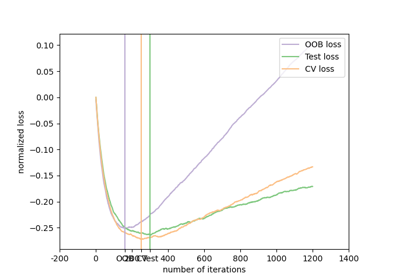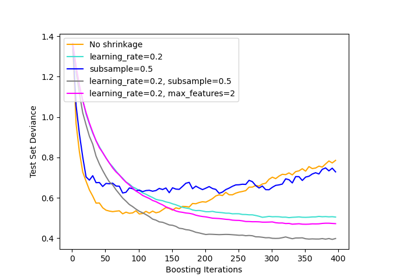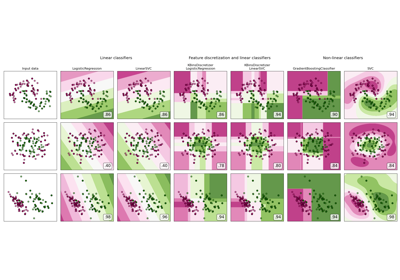# sklearn.ensemble.GradientBoostingClassifier¶

class sklearn.ensemble.GradientBoostingClassifier(*, loss='log_loss', learning_rate=0.1, n_estimators=100, subsample=1.0, criterion='friedman_mse', min_samples_split=2, min_samples_leaf=1, min_weight_fraction_leaf=0.0, max_depth=3, min_impurity_decrease=0.0, init=None, random_state=None, max_features=None, verbose=0, max_leaf_nodes=None, warm_start=False, validation_fraction=0.1, n_iter_no_change=None, tol=0.0001, ccp_alpha=0.0)[source]

This algorithm builds an additive model in a forward stage-wise fashion; it allows for the optimization of arbitrary differentiable loss functions. In each stage n_classes_ regression trees are fit on the negative gradient of the loss function, e.g. binary or multiclass log loss. Binary classification is a special case where only a single regression tree is induced.

sklearn.ensemble.HistGradientBoostingClassifier is a much faster variant of this algorithm for intermediate datasets (n_samples >= 10_000).

Read more in the User Guide.

Parameters:
loss{‘log_loss’, ‘deviance’, ‘exponential’}, default=’log_loss’

The loss function to be optimized. ‘log_loss’ refers to binomial and multinomial deviance, the same as used in logistic regression. It is a good choice for classification with probabilistic outputs. For loss ‘exponential’, gradient boosting recovers the AdaBoost algorithm.

Deprecated since version 1.1: The loss ‘deviance’ was deprecated in v1.1 and will be removed in version 1.3. Use loss='log_loss' which is equivalent.

learning_ratefloat, default=0.1

Learning rate shrinks the contribution of each tree by learning_rate. There is a trade-off between learning_rate and n_estimators. Values must be in the range (0.0, inf).

n_estimatorsint, default=100

The number of boosting stages to perform. Gradient boosting is fairly robust to over-fitting so a large number usually results in better performance. Values must be in the range [1, inf).

subsamplefloat, default=1.0

The fraction of samples to be used for fitting the individual base learners. If smaller than 1.0 this results in Stochastic Gradient Boosting. subsample interacts with the parameter n_estimators. Choosing subsample < 1.0 leads to a reduction of variance and an increase in bias. Values must be in the range (0.0, 1.0].

criterion{‘friedman_mse’, ‘squared_error’, ‘mse’}, default=’friedman_mse’

The function to measure the quality of a split. Supported criteria are ‘friedman_mse’ for the mean squared error with improvement score by Friedman, ‘squared_error’ for mean squared error. The default value of ‘friedman_mse’ is generally the best as it can provide a better approximation in some cases.

New in version 0.18.

Deprecated since version 1.0: Criterion ‘mse’ was deprecated in v1.0 and will be removed in version 1.2. Use criterion='squared_error' which is equivalent.

min_samples_splitint or float, default=2

The minimum number of samples required to split an internal node:

• If int, values must be in the range [2, inf).

• If float, values must be in the range (0.0, 1.0] and min_samples_split will be ceil(min_samples_split * n_samples).

Changed in version 0.18: Added float values for fractions.

min_samples_leafint or float, default=1

The minimum number of samples required to be at a leaf node. A split point at any depth will only be considered if it leaves at least min_samples_leaf training samples in each of the left and right branches. This may have the effect of smoothing the model, especially in regression.

• If int, values must be in the range [1, inf).

• If float, values must be in the range (0.0, 1.0] and min_samples_leaf will be ceil(min_samples_leaf * n_samples).

Changed in version 0.18: Added float values for fractions.

min_weight_fraction_leaffloat, default=0.0

The minimum weighted fraction of the sum total of weights (of all the input samples) required to be at a leaf node. Samples have equal weight when sample_weight is not provided. Values must be in the range [0.0, 0.5].

max_depthint, default=3

The maximum depth of the individual regression estimators. The maximum depth limits the number of nodes in the tree. Tune this parameter for best performance; the best value depends on the interaction of the input variables. Values must be in the range [1, inf).

min_impurity_decreasefloat, default=0.0

A node will be split if this split induces a decrease of the impurity greater than or equal to this value. Values must be in the range [0.0, inf).

The weighted impurity decrease equation is the following:

N_t / N * (impurity - N_t_R / N_t * right_impurity
- N_t_L / N_t * left_impurity)


where N is the total number of samples, N_t is the number of samples at the current node, N_t_L is the number of samples in the left child, and N_t_R is the number of samples in the right child.

N, N_t, N_t_R and N_t_L all refer to the weighted sum, if sample_weight is passed.

New in version 0.19.

initestimator or ‘zero’, default=None

An estimator object that is used to compute the initial predictions. init has to provide fit and predict_proba. If ‘zero’, the initial raw predictions are set to zero. By default, a DummyEstimator predicting the classes priors is used.

random_stateint, RandomState instance or None, default=None

Controls the random seed given to each Tree estimator at each boosting iteration. In addition, it controls the random permutation of the features at each split (see Notes for more details). It also controls the random splitting of the training data to obtain a validation set if n_iter_no_change is not None. Pass an int for reproducible output across multiple function calls. See Glossary.

max_features{‘auto’, ‘sqrt’, ‘log2’}, int or float, default=None

The number of features to consider when looking for the best split:

• If int, values must be in the range [1, inf).

• If float, values must be in the range (0.0, 1.0] and the features considered at each split will be max(1, int(max_features * n_features_in_)).

• If ‘auto’, then max_features=sqrt(n_features).

• If ‘sqrt’, then max_features=sqrt(n_features).

• If ‘log2’, then max_features=log2(n_features).

• If None, then max_features=n_features.

Choosing max_features < n_features leads to a reduction of variance and an increase in bias.

Note: the search for a split does not stop until at least one valid partition of the node samples is found, even if it requires to effectively inspect more than max_features features.

verboseint, default=0

Enable verbose output. If 1 then it prints progress and performance once in a while (the more trees the lower the frequency). If greater than 1 then it prints progress and performance for every tree. Values must be in the range [0, inf).

max_leaf_nodesint, default=None

Grow trees with max_leaf_nodes in best-first fashion. Best nodes are defined as relative reduction in impurity. Values must be in the range [2, inf). If None, then unlimited number of leaf nodes.

warm_startbool, default=False

When set to True, reuse the solution of the previous call to fit and add more estimators to the ensemble, otherwise, just erase the previous solution. See the Glossary.

validation_fractionfloat, default=0.1

The proportion of training data to set aside as validation set for early stopping. Values must be in the range (0.0, 1.0). Only used if n_iter_no_change is set to an integer.

New in version 0.20.

n_iter_no_changeint, default=None

n_iter_no_change is used to decide if early stopping will be used to terminate training when validation score is not improving. By default it is set to None to disable early stopping. If set to a number, it will set aside validation_fraction size of the training data as validation and terminate training when validation score is not improving in all of the previous n_iter_no_change numbers of iterations. The split is stratified. Values must be in the range [1, inf).

New in version 0.20.

tolfloat, default=1e-4

Tolerance for the early stopping. When the loss is not improving by at least tol for n_iter_no_change iterations (if set to a number), the training stops. Values must be in the range (0.0, inf).

New in version 0.20.

ccp_alphanon-negative float, default=0.0

Complexity parameter used for Minimal Cost-Complexity Pruning. The subtree with the largest cost complexity that is smaller than ccp_alpha will be chosen. By default, no pruning is performed. Values must be in the range [0.0, inf). See Minimal Cost-Complexity Pruning for details.

New in version 0.22.

Attributes:
n_estimators_int

The number of estimators as selected by early stopping (if n_iter_no_change is specified). Otherwise it is set to n_estimators.

New in version 0.20.

feature_importances_ndarray of shape (n_features,)

The impurity-based feature importances.

oob_improvement_ndarray of shape (n_estimators,)

The improvement in loss (= deviance) on the out-of-bag samples relative to the previous iteration. oob_improvement_ is the improvement in loss of the first stage over the init estimator. Only available if subsample < 1.0

train_score_ndarray of shape (n_estimators,)

The i-th score train_score_[i] is the deviance (= loss) of the model at iteration i on the in-bag sample. If subsample == 1 this is the deviance on the training data.

loss_LossFunction

DEPRECATED: Attribute loss_ was deprecated in version 1.1 and will be removed in 1.3.

init_estimator

The estimator that provides the initial predictions. Set via the init argument or loss.init_estimator.

estimators_ndarray of DecisionTreeRegressor of shape (n_estimators, loss_.K)

The collection of fitted sub-estimators. loss_.K is 1 for binary classification, otherwise n_classes.

classes_ndarray of shape (n_classes,)

The classes labels.

n_features_int

DEPRECATED: Attribute n_features_ was deprecated in version 1.0 and will be removed in 1.2.

n_features_in_int

Number of features seen during fit.

New in version 0.24.

feature_names_in_ndarray of shape (n_features_in_,)

Names of features seen during fit. Defined only when X has feature names that are all strings.

New in version 1.0.

n_classes_int

The number of classes.

max_features_int

The inferred value of max_features.

HistGradientBoostingClassifier

sklearn.tree.DecisionTreeClassifier

A decision tree classifier.

RandomForestClassifier

A meta-estimator that fits a number of decision tree classifiers on various sub-samples of the dataset and uses averaging to improve the predictive accuracy and control over-fitting.

AdaBoostClassifier

A meta-estimator that begins by fitting a classifier on the original dataset and then fits additional copies of the classifier on the same dataset where the weights of incorrectly classified instances are adjusted such that subsequent classifiers focus more on difficult cases.

Notes

The features are always randomly permuted at each split. Therefore, the best found split may vary, even with the same training data and max_features=n_features, if the improvement of the criterion is identical for several splits enumerated during the search of the best split. To obtain a deterministic behaviour during fitting, random_state has to be fixed.

References

J. Friedman, Greedy Function Approximation: A Gradient Boosting Machine, The Annals of Statistics, Vol. 29, No. 5, 2001.

1. Friedman, Stochastic Gradient Boosting, 1999

T. Hastie, R. Tibshirani and J. Friedman. Elements of Statistical Learning Ed. 2, Springer, 2009.

Examples

The following example shows how to fit a gradient boosting classifier with 100 decision stumps as weak learners.

>>> from sklearn.datasets import make_hastie_10_2

>>> X, y = make_hastie_10_2(random_state=0)
>>> X_train, X_test = X[:2000], X[2000:]
>>> y_train, y_test = y[:2000], y[2000:]

>>> clf = GradientBoostingClassifier(n_estimators=100, learning_rate=1.0,
...     max_depth=1, random_state=0).fit(X_train, y_train)
>>> clf.score(X_test, y_test)
0.913...


Methods

 Apply trees in the ensemble to X, return leaf indices. Compute the decision function of X. fit(X, y[, sample_weight, monitor]) Fit the gradient boosting model. get_params([deep]) Get parameters for this estimator. Predict class for X. Predict class log-probabilities for X. Predict class probabilities for X. score(X, y[, sample_weight]) Return the mean accuracy on the given test data and labels. set_params(**params) Set the parameters of this estimator. Compute decision function of X for each iteration. Predict class at each stage for X. Predict class probabilities at each stage for X.
apply(X)[source]

Apply trees in the ensemble to X, return leaf indices.

New in version 0.17.

Parameters:
X{array-like, sparse matrix} of shape (n_samples, n_features)

The input samples. Internally, its dtype will be converted to dtype=np.float32. If a sparse matrix is provided, it will be converted to a sparse csr_matrix.

Returns:
X_leavesarray-like of shape (n_samples, n_estimators, n_classes)

For each datapoint x in X and for each tree in the ensemble, return the index of the leaf x ends up in each estimator. In the case of binary classification n_classes is 1.

decision_function(X)[source]

Compute the decision function of X.

Parameters:
X{array-like, sparse matrix} of shape (n_samples, n_features)

The input samples. Internally, it will be converted to dtype=np.float32 and if a sparse matrix is provided to a sparse csr_matrix.

Returns:
scorendarray of shape (n_samples, n_classes) or (n_samples,)

The decision function of the input samples, which corresponds to the raw values predicted from the trees of the ensemble . The order of the classes corresponds to that in the attribute classes_. Regression and binary classification produce an array of shape (n_samples,).

property feature_importances_

The impurity-based feature importances.

The higher, the more important the feature. The importance of a feature is computed as the (normalized) total reduction of the criterion brought by that feature. It is also known as the Gini importance.

Warning: impurity-based feature importances can be misleading for high cardinality features (many unique values). See sklearn.inspection.permutation_importance as an alternative.

Returns:
feature_importances_ndarray of shape (n_features,)

The values of this array sum to 1, unless all trees are single node trees consisting of only the root node, in which case it will be an array of zeros.

fit(X, y, sample_weight=None, monitor=None)[source]

Parameters:
X{array-like, sparse matrix} of shape (n_samples, n_features)

The input samples. Internally, it will be converted to dtype=np.float32 and if a sparse matrix is provided to a sparse csr_matrix.

yarray-like of shape (n_samples,)

Target values (strings or integers in classification, real numbers in regression) For classification, labels must correspond to classes.

sample_weightarray-like of shape (n_samples,), default=None

Sample weights. If None, then samples are equally weighted. Splits that would create child nodes with net zero or negative weight are ignored while searching for a split in each node. In the case of classification, splits are also ignored if they would result in any single class carrying a negative weight in either child node.

monitorcallable, default=None

The monitor is called after each iteration with the current iteration, a reference to the estimator and the local variables of _fit_stages as keyword arguments callable(i, self, locals()). If the callable returns True the fitting procedure is stopped. The monitor can be used for various things such as computing held-out estimates, early stopping, model introspect, and snapshoting.

Returns:
selfobject

Fitted estimator.

get_params(deep=True)[source]

Get parameters for this estimator.

Parameters:
deepbool, default=True

If True, will return the parameters for this estimator and contained subobjects that are estimators.

Returns:
paramsdict

Parameter names mapped to their values.

property loss_

DEPRECATED: Attribute loss_ was deprecated in version 1.1 and will be removed in 1.3.

property n_features_

DEPRECATED: Attribute n_features_ was deprecated in version 1.0 and will be removed in 1.2. Use n_features_in_ instead.

predict(X)[source]

Predict class for X.

Parameters:
X{array-like, sparse matrix} of shape (n_samples, n_features)

The input samples. Internally, it will be converted to dtype=np.float32 and if a sparse matrix is provided to a sparse csr_matrix.

Returns:
yndarray of shape (n_samples,)

The predicted values.

predict_log_proba(X)[source]

Predict class log-probabilities for X.

Parameters:
X{array-like, sparse matrix} of shape (n_samples, n_features)

The input samples. Internally, it will be converted to dtype=np.float32 and if a sparse matrix is provided to a sparse csr_matrix.

Returns:
pndarray of shape (n_samples, n_classes)

The class log-probabilities of the input samples. The order of the classes corresponds to that in the attribute classes_.

Raises:
AttributeError

If the loss does not support probabilities.

predict_proba(X)[source]

Predict class probabilities for X.

Parameters:
X{array-like, sparse matrix} of shape (n_samples, n_features)

The input samples. Internally, it will be converted to dtype=np.float32 and if a sparse matrix is provided to a sparse csr_matrix.

Returns:
pndarray of shape (n_samples, n_classes)

The class probabilities of the input samples. The order of the classes corresponds to that in the attribute classes_.

Raises:
AttributeError

If the loss does not support probabilities.

score(X, y, sample_weight=None)[source]

Return the mean accuracy on the given test data and labels.

In multi-label classification, this is the subset accuracy which is a harsh metric since you require for each sample that each label set be correctly predicted.

Parameters:
Xarray-like of shape (n_samples, n_features)

Test samples.

yarray-like of shape (n_samples,) or (n_samples, n_outputs)

True labels for X.

sample_weightarray-like of shape (n_samples,), default=None

Sample weights.

Returns:
scorefloat

Mean accuracy of self.predict(X) wrt. y.

set_params(**params)[source]

Set the parameters of this estimator.

The method works on simple estimators as well as on nested objects (such as Pipeline). The latter have parameters of the form <component>__<parameter> so that it’s possible to update each component of a nested object.

Parameters:
**paramsdict

Estimator parameters.

Returns:
selfestimator instance

Estimator instance.

staged_decision_function(X)[source]

Compute decision function of X for each iteration.

This method allows monitoring (i.e. determine error on testing set) after each stage.

Parameters:
X{array-like, sparse matrix} of shape (n_samples, n_features)

The input samples. Internally, it will be converted to dtype=np.float32 and if a sparse matrix is provided to a sparse csr_matrix.

Yields:
scoregenerator of ndarray of shape (n_samples, k)

The decision function of the input samples, which corresponds to the raw values predicted from the trees of the ensemble . The classes corresponds to that in the attribute classes_. Regression and binary classification are special cases with k == 1, otherwise k==n_classes.

staged_predict(X)[source]

Predict class at each stage for X.

This method allows monitoring (i.e. determine error on testing set) after each stage.

Parameters:
X{array-like, sparse matrix} of shape (n_samples, n_features)

The input samples. Internally, it will be converted to dtype=np.float32 and if a sparse matrix is provided to a sparse csr_matrix.

Yields:
ygenerator of ndarray of shape (n_samples,)

The predicted value of the input samples.

staged_predict_proba(X)[source]

Predict class probabilities at each stage for X.

This method allows monitoring (i.e. determine error on testing set) after each stage.

Parameters:
X{array-like, sparse matrix} of shape (n_samples, n_features)

The input samples. Internally, it will be converted to dtype=np.float32 and if a sparse matrix is provided to a sparse csr_matrix.

Yields:
ygenerator of ndarray of shape (n_samples,)

The predicted value of the input samples.

## Examples using sklearn.ensemble.GradientBoostingClassifier¶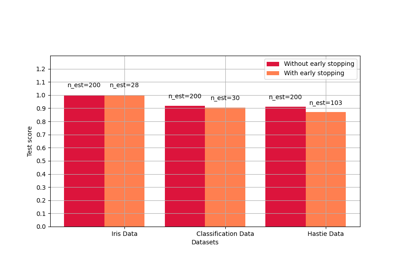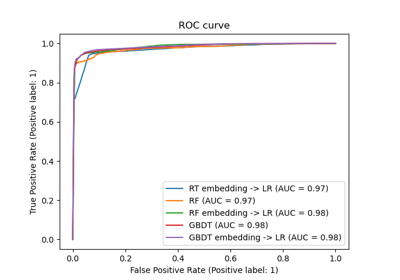Feature transformations with ensembles of trees

Feature transformations with ensembles of trees